# Linear Regression With Ordinary Least Squared Method and Gradient Descent From Scratch In Python.

## Linear regression is modeling the linear relationships between real-valued predictors and target variables. We find the function that best representative of the data.

Introduction

Linear regression is the first model we learn about regression analysis since high school. We are looking for regression line that fit the samples drawn from population with the most commonly used statistical method, ordinary least square regression (OLS). Weestimate model parameters. However, estimates functions get complex as we have more independent variables to be included in the model. Gradient descent (GD)is another option that is widely applied to a range of models training. It is simpler to implement for linear regression model.

Here, for simplicity, we are trying to build a linear regression from scratch for simple linear regression.

OLS

Ordinary least square method is non-iterative method to fit a model by seeking to minimize sum of squared errors. There is a list of assumptions to satisfy when we are applying OLS.

Assumption:

• Function must be linear in parameters and error term.
• Error terms are independent with each other and all independent variables.
• Error terms are normally distributed with mean of zero and constant variance.
• No independent variables are perfectly correlated.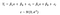Fig 1: OLS regression function and error term distribution.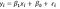Fig 2: Simple linear regression function.

Estimates of coefficients for simple linear regression are derived as below:

1. Compute sum of square error, SSE.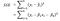Fig 3: Sum of Squared Error.

2. Differentiate with respect to parameters.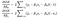Fig 4: Differential equations.

3. Let differential equation equal to zero. Solve the simultaneous equation to get estimates of parameters.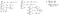Fig 5:Derivation of estimates for parameters.

The process above gets lengthy and complicated as we have more independent variables included, and hence more estimate functions to be derived.

OLS from Scratch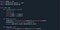Fig 6: Creating a class for linear regression with OLS.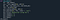Fig 7: Load data and run OLS.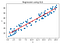Fig 8: Regression with OLS.

Objective Function / Loss function

Loss function is the cost function associated with error in prediction. Error is difference between our predictions and true values. Often, we square the error for ease of derivatives computation. Loss function, mean squared error will be applied in gradient descent method below.Fig 9: Mean Squared Error.

Gradient Descent

This is a popular optimization method. We are working iterative to find best coefficients for regression line, having the minimum loss. The logic behind gradient descent is like when a ball rolling down a curve. To reach the bottom, it should move in opposite direction to the slope. The scenario is illustrated below.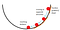Fig 10: Illustration of gradient descent idea.

What is the step size to be taken to ensure we do not miss the bottom? This will be the challenge in GD method. When the step size is too large, we miss the destination. When the step size is too small, we take too long to the point.

1. Compute gradient of loss function.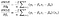Fig 11: Gradient of mean squared error with respect to parameters.

2. Selecting appropriate learning rate. Randomly select parameters of linear regression function.

3. Update the parameters.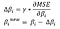Fig 12: line 1 is the parameter update size.

4. Repeat the process until the loss is within our acceptance level or parameters converges.

Model from Scratch by Python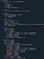Fig 13: Creating a class for linear regression with gradient descent.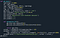Fig 14: Loading data and start training.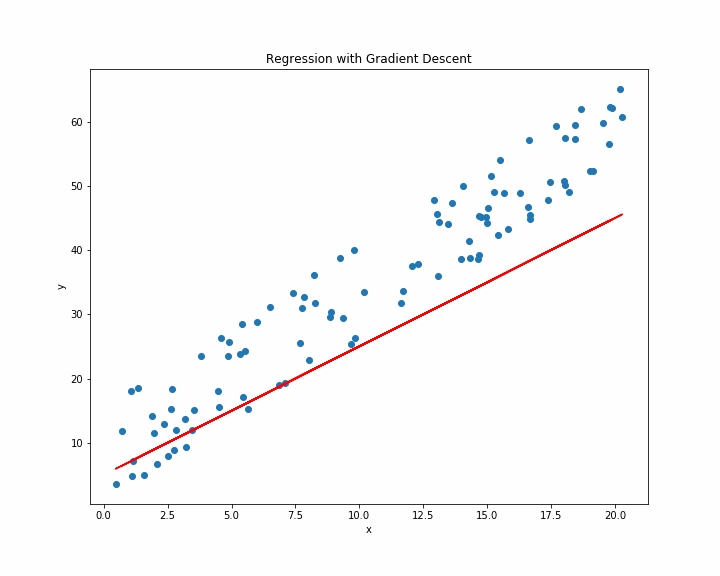Fig 15: Visualizing gradient descent updating process.

Summary

Looking at gradient descent visualization above, the degree at which the fitted line rotates and shifts appears to slow down as it approaches the final result. This is similar to the ball rolling illustration, as the ball approaches bottom, gradient decreases, and hence update size (delta in Fig 13) decreases. As discussed, OLS is a single run where information is substituting into equation derived to get estimates of parameter directly, while GD is running iterative until it arrived at the best result satisfying required condition. Obviously, OLS will become tougher to apply as features dimension increases.

## The Startup

Get smarter at building your thing. Join The Startup’s +785K followers.

## Sign up for Top 10 Stories

### By The Startup

Get smarter at building your thing. Subscribe to receive The Startup's top 10 most read stories — delivered straight into your inbox, once a week. Take a look.

By signing up, you will create a Medium account if you don’t already have one. Review our Privacy Policy for more information about our privacy practices.

Check your inbox
Medium sent you an email at to complete your subscription.

## The Startup

Get smarter at building your thing. Follow to join The Startup’s +8 million monthly readers & +785K followers.## The Startup

Get smarter at building your thing. Follow to join The Startup’s +8 million monthly readers & +785K followers.

Medium is an open platform where 170 million readers come to find insightful and dynamic thinking. Here, expert and undiscovered voices alike dive into the heart of any topic and bring new ideas to the surface. Learn more

Follow the writers, publications, and topics that matter to you, and you’ll see them on your homepage and in your inbox. Explore

If you have a story to tell, knowledge to share, or a perspective to offer — welcome home. It’s easy and free to post your thinking on any topic. Write on Medium